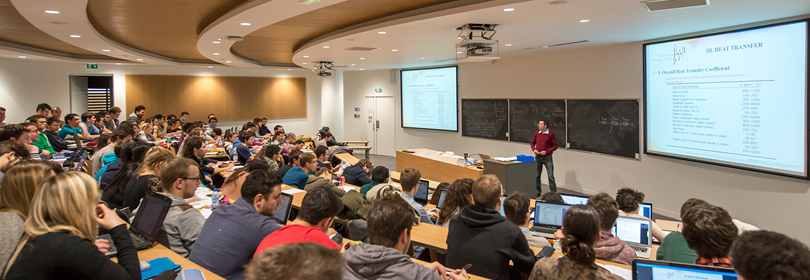Our engineering & master degrees
Ecole nationale supérieure de l'Énergie, l'Eau et l'Environnement
-
Engineering school in energy, water and environment# Electrical Engineering - 3EUS1ENE

A+Augmenter la taille du texteA-Réduire la taille du texteImprimer le documentEnvoyer cette page par mail cet article Facebook Twitter Linked In
• #### Number of hours

• Lectures : 24.0
• Tutorials : 20.0
• Laboratory works : 16.0
• Projects : -
• Internship : -
ECTS : 5.0

### Goals

This course is an introduction to electric power system and electric machinery. This field has become increasingly important to transmit and transform energy in industrial and transportation uses. The course introduces the entire electric power system from single phase and three phase circuits to rotating electric machinery via the transformation of electric energy through power electronics, or static conversion. The course focuses on the study of the various components by giving a simple model often sufficient for a first approach. We will place deliberately from the viewpoint of the user, and not from the viewpoint of the designer. Some elements on electrical grids are given.

Key words:
Single phase, three-phase circuit, transformer, rotating electric machinery, synchronous machine, induction machine, power electronics, static converter, chopper, inverter, rectifier.

Course Learning Outcomes
Have an overview of electric power systems including generation, transmission, distribution and usage.
Perform power engineering circuit calculations (phasor diagram, powers (complex, real and reactive), power-factor correction, equivalent series and parallel circuits). Three-phase AC balanced circuit analysis, concepts and terminology (star/delta…), single-phase equivalent circuits.
Understand transformers: magnetostatics and electromagnetics (magnetic circuits, magnetic field, magnetic flux density, magnetic materials (permeability saturation, BH loops, iron loss) inductances, Faraday’s and Lenz law) transformers: ideal and practical transformers (equivalent circuit, voltage drop, efficiency)
Understand the operating principles and analysis of DC machines seen as a perfect electric machine. Introduce the control of electric machines (starting of a DC motor as example).
Understand the operating principles and analysis of synchronous machines and to a lesser degree induction machines (equivalent circuits and performance prediction).
Understand the power electronic principles for the control and conversion of electric power (switching) and the main static converters (chopper (DC to DC)), inverter (DC to AC)) and rectifier (AC to DC)).
Develop a practical understanding by performing a series of laboratory experiments.

Bibliography, Textbook
“Energie électrique”, P. Tixador, Ense3 document.
“Electric machinery and power system - Fundamentals”, S. J. Chapman, Mc Graw Hill, 2002, ISBN 0-07-122620-6.
“Électrotechnique”, Théodore Wildi, Gilbert Sybille, Editeur : De Boeck, ISBN-10 : 2804148920, 2005.
“Eléments de Génie Electrique - Connaissances de base et machines statiques”, M. Ivanes, R. Perret, Hermès, Paris, 1994.
“Electrotechnique industrielle”, G. Séguier, F. Notelet, Technique et documentation, Paris, 3ème édition, 2006.

Content

• Energy and electric energy
• Electric energy - General points
• Single phase circuit, real and reactive powers
• Three-phase circuit
• Magnetic circuits and inductances
• Transformers
• Electromechanical energy conversion
• DC machine as ideal machine to control
• Rotating magnetic field and study method of AC machines
• Synchronous machines
• Induction machine
• Power electronics - Introduction and overview
• Chopper - DC-DC converter
• Inverter - DC-AC Converter
• Rectifier - AC-DC converter

Prerequisites

electric circuit (phasor diagram, complex number and impedance)

Tests

CC 40% + CT 60%

Calendar

The course exists in the following branches:

see the course schedule for 2019-2020

Course language(s):